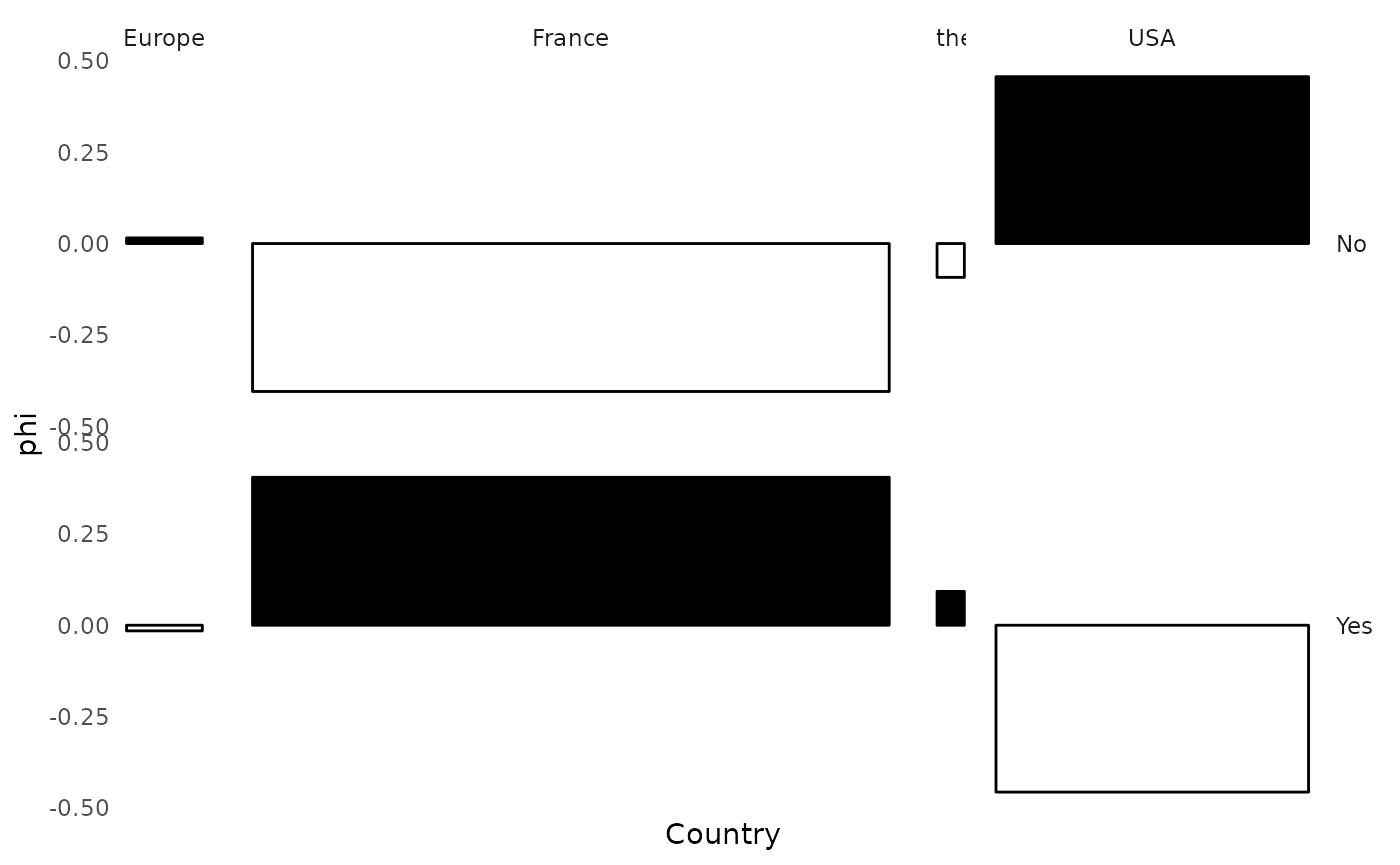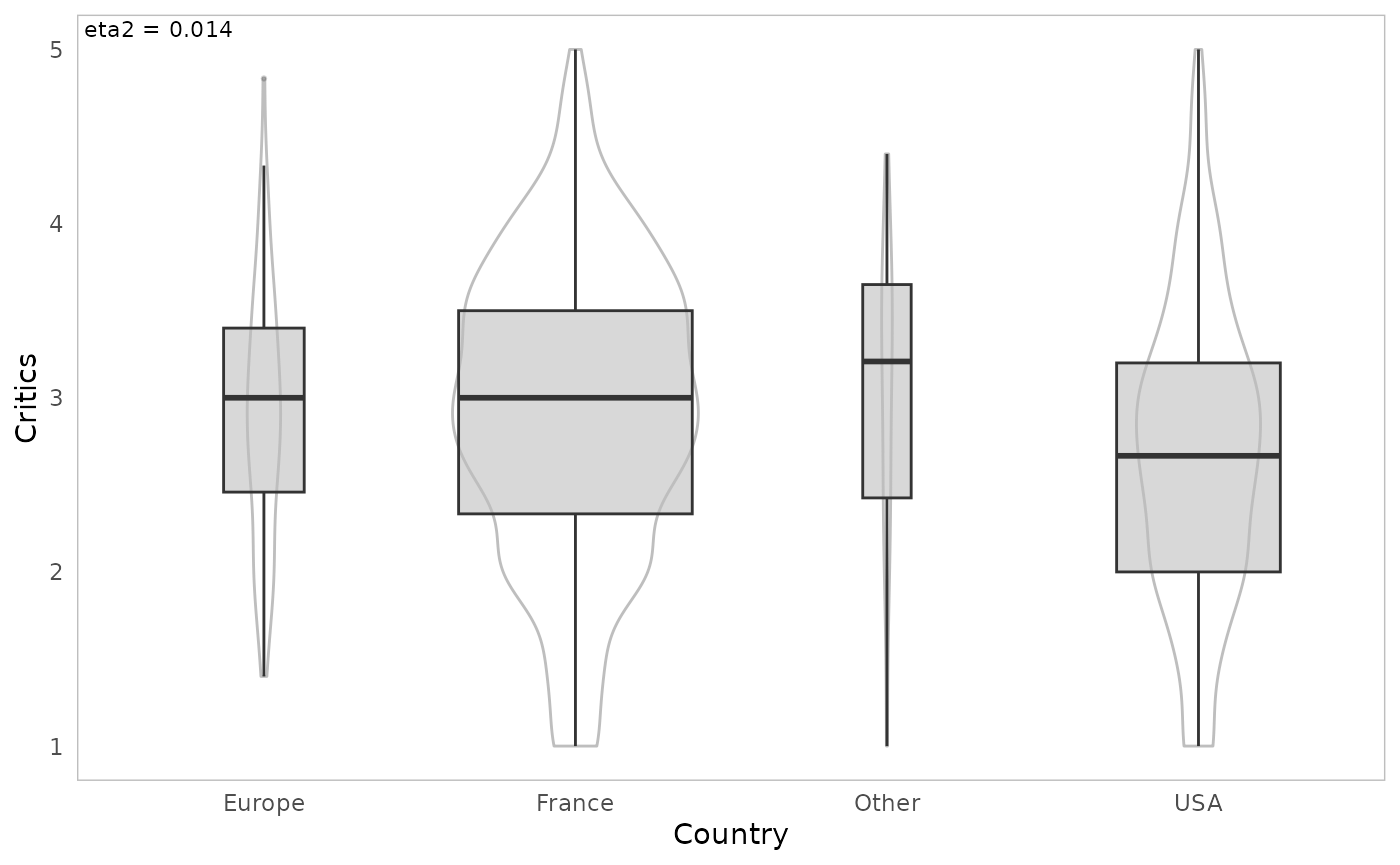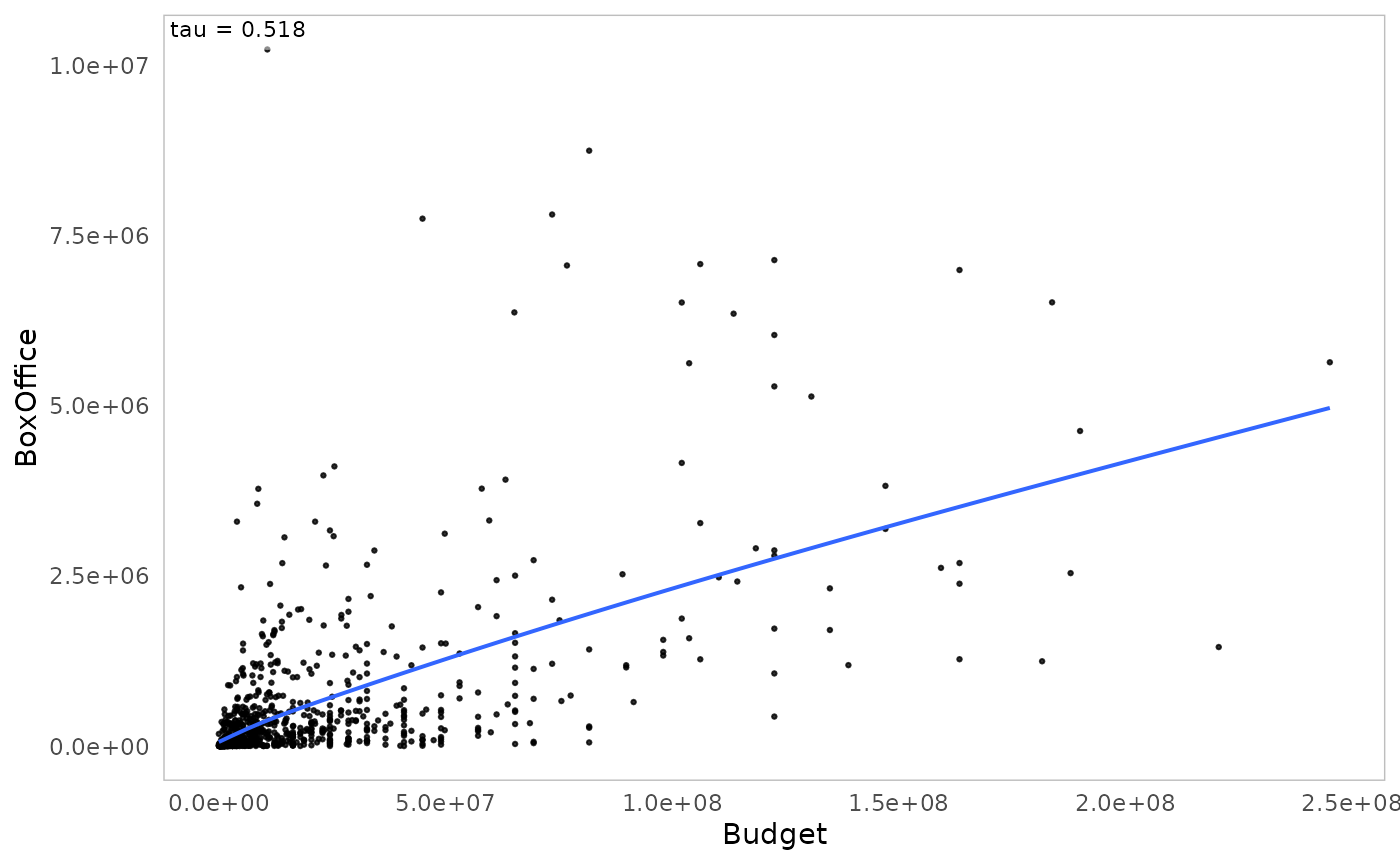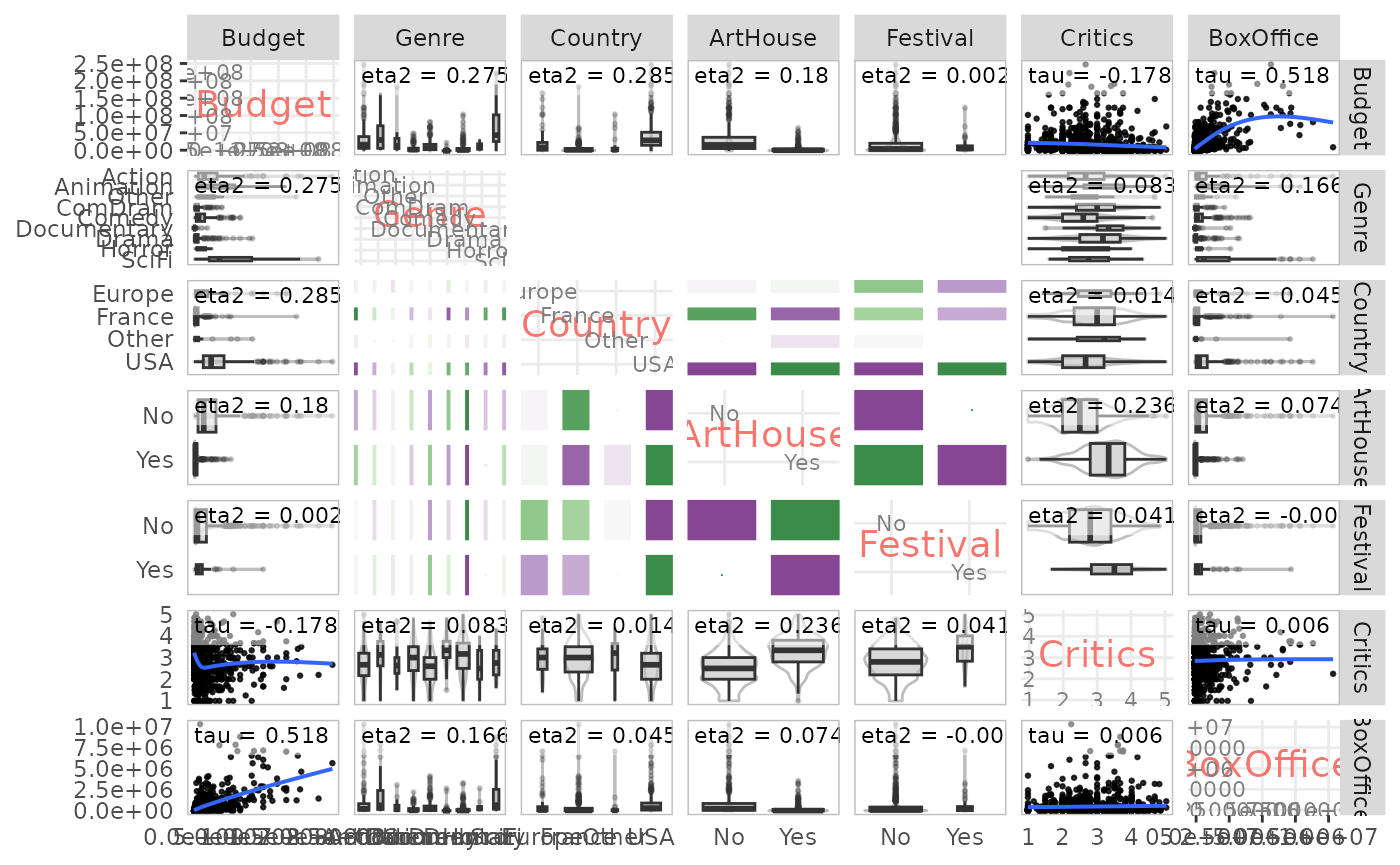GDAtools package provides some functions dedicated to the description of statistical associations between variables. They are based on effect size measures (also called association measures).

All these measures are built from simple concepts (correlations, proportion of variance explained), they are bounded (between -1 and 1 or between 0 and 1) and are not sensitive to the number of observations.

The measures of global association are the following.

• For the relationship between two categorical variables: the Cramér’s V which, unlike the chi-square, for example, is not sensitive to the number of observations or the number of categories of the variables. It varies between 0 (no association) and 1 (perfect association). Squared, it can be interpreted as the share of variation shared between two variables.

• For the relationship between two numerical variables: Kendall’s (tau) or Spearman’s (rho) rank correlations, which detect monotonic relationships between variables, and not only linear ones as is the case with Pearson’s linear correlation. They vary between -1 and 1. An absolute value of 0 indicates no association, an absolute value of 1 a perfect association. The sign indicates the direction of the relationship.

• For the relationship between a categorical variable and a numerical variable: the square of the correlation ratio (eta²). It expresses the proportion of the variance of the numerical variable “explained” by the categorical variable and varies between 0 and 1.

In addition to measures of global association, we also use measures of local association, i.e. at the level of the categories of the variables.

• For the relationship between two categorical variables: the phi coefficient measures the attraction or repulsion in a cell of a contingency table. It varies between -1 and 1. An absolute value of 0 indicates an absence of association, an absolute value of 1 a perfect association. There is attraction if the sign is positive, repulsion if the sign is negative. Squared, phi is interpreted as the proportion of variance shared by the two binary variables associated with the categories studied. Unlike the test value, phi is not sensitive to the sample size.

• For the relationship between a categorical variable and a numerical variable: the point biserial correlation measures the magnitude of the difference between the means of the numerical variable according to whether or not one belongs to the category studied. It varies between -1 and 1. An absolute value of 0 indicates no association, an absolute value of 1 a perfect association. The sign indicates the direction of the relationship. When squared, point biserial correlation can be interpreted as the proportion of variance of the numerical variable “explained” by the category of the categorical variable.

Note that if we code the categories of the categorical variables as binary variables with values of 0 or 1, the phi coefficient and the point biserial correlation are equivalent to Pearson’s correlation coefficient.

For more details on these effect size measurements, see: Rakotomalala R., « Comprendre la taille d’effet (effect size) »

In some functions of GDAtools, association measures can be completed by permutation tests, which are part of combinatorial inference and constitute a nonparametric alternative to the significance tests of frequentist inference. A permutation test is performed in several steps.

1. A measure of association between the two variables under study is computed.

2. The same measure of association is calculated from a “permuted” version of the data, i.e. by randomly “mixing” the values of one of the variables, in order to “break” the relationship between the variables.

3. Repeat step 2 a large number of times. This gives an empirical distribution (as opposed to the use of a theoretical distribution by frequentist inference) of the measure of association under the H0 hypothesis of no relationship between the two variables.

4. The result of step 1 is compared with the distribution obtained in 3. The p-value of the permutation test is the proportion of values of the H0 distribution that are more extreme than the measure of association observed in 1.

If all possible permutations are performed, the permutation test is called “exact”. In practice, the computation time required is often too important and only a part of the possible permutations is performed, resulting in an “approximate” test. In the following examples, the number of permutations is set to 100 to reduce the computation time, but it is advisable to increase this number to obtain more accurate and reliable results (for example nperm=1000).

To illustrate the statistical association analysis functions of GDAtools, we will use data on cinema. This is a sample of 1000 films released in France in the 2000s, for which we know the budget, the genre, the country of origin, the “art et essai” label, the selection in a festival (Cannes, Berlin or Venice), the average rating of intellectual critics (according to Allociné) and the number of admissions. Some of these variables are numerical, others are categorical.

library(GDAtools)
data(Movies)
str(Movies)
'data.frame':   1000 obs. of  7 variables:
$Budget : num 3.10e+07 4.88e+06 3.50e+06 1.63e+08 2.17e+07 ...$ Genre    : Factor w/ 9 levels "Action","Animation",..: 1 5 7 1 7 5 1 7 5 7 ...
$Country : Factor w/ 4 levels "Europe","France",..: 4 2 2 1 2 2 4 4 2 4 ...$ ArtHouse : Factor w/ 2 levels "No","Yes": 1 1 2 1 2 1 1 1 1 1 ...
$Festival : Factor w/ 2 levels "No","Yes": 1 1 1 1 1 1 1 1 1 1 ...$ Critics  : num  3 1 3.75 3.75 3.6 2.75 1 1 1 3 ...
$BoxOffice: num 1013509 24241 39376 6996996 493416 ... # Relationship between two variables The package offers several functions to study the statistical relationship between two variables, depending on the nature (categorical or numerical) of these variables. ### Two categorical variables The function assoc_twocat computes : • the contingency table (numbers) • the percentages, the row-percentages and the column-percentages • the theoretical numbers, i.e. in a situation of independence • the chi-square • the Cramér’s V and the p-value of the corresponding permutation test • the Pearson residuals • the phi coefficients and the p-values of the corresponding permutation tests • the global and local PEMs (Percentage of Maximum Deviation from Independence, see Cibois 1993) • a summary table of these results assoc.twocat(Movies$Country, Movies$ArtHouse, nperm=100) $freq
No  Yes  Sum
Europe   39   33   72
France  212  393  605
Other     6   20   26
USA     257   40  297
Sum     514  486 1000

$prop No Yes Sum Europe 3.9 3.3 7.2 France 21.2 39.3 60.5 Other 0.6 2.0 2.6 USA 25.7 4.0 29.7 Sum 51.4 48.6 100.0$rprop
No      Yes Sum
Europe 54.16667 45.83333 100
France 35.04132 64.95868 100
Other  23.07692 76.92308 100
USA    86.53199 13.46801 100
Sum    51.40000 48.60000 100

$cprop No Yes Sum Europe 7.587549 6.790123 7.2 France 41.245136 80.864198 60.5 Other 1.167315 4.115226 2.6 USA 50.000000 8.230453 29.7 Sum 100.000000 100.000000 100.0$expected
No     Yes
Europe  37.008  34.992
France 310.970 294.030
Other   13.364  12.636
USA    152.658 144.342

$chi.squared  220.1263$cramer.v
 0.4691762

$permutation.pvalue  0$pearson.residuals
No        Yes
Europe  0.3274474 -0.3367479
France -5.6123445  5.7717531
Other  -2.0143992  2.0716146
USA     8.4449945 -8.6848595

$phi No Yes Europe 0.01541876 -0.01541876 France -0.40506773 0.40506773 Other -0.09258656 0.09258656 USA 0.45688150 -0.45688150$phi.perm.pval
No          Yes
Europe 2.758473e-01 2.758473e-01
France 7.924539e-47 0.000000e+00
Other  2.033023e-03 2.033023e-03
USA    0.000000e+00 8.201030e-51

$gather Var1 Var2 Freq prop rprop cprop expected std.residuals phi perm.pval 1 Europe No 39 0.039 0.5416667 0.07587549 37.008 0.3274474 0.01541876 2.758473e-01 2 France No 212 0.212 0.3504132 0.41245136 310.970 -5.6123445 -0.40506773 7.924539e-47 3 Other No 6 0.006 0.2307692 0.01167315 13.364 -2.0143992 -0.09258656 2.033023e-03 4 USA No 257 0.257 0.8653199 0.50000000 152.658 8.4449945 0.45688150 0.000000e+00 5 Europe Yes 33 0.033 0.4583333 0.06790123 34.992 -0.3367479 -0.01541876 2.758473e-01 6 France Yes 393 0.393 0.6495868 0.80864198 294.030 5.7717531 0.40506773 0.000000e+00 7 Other Yes 20 0.020 0.7692308 0.04115226 12.636 2.0716146 0.09258656 2.033023e-03 [ reached 'max' / getOption("max.print") -- omitted 1 rows ] The function ggassoc_crosstab presents the contingency table in graphical form, with rectangles whose area corresponds to the numbers and whose color gradient corresponds to the attractions/repulsions (phi coefficients). The Cramér’s V can be displayed in a corner of the graph. Here, the “art et essai” label is clearly over-represented among French films and under-represented among American films. ggassoc_crosstab(Movies, ggplot2::aes(x=Country, y=ArtHouse), max.phi=0.8)The function ggassoc_phiplot proposes another way to represent the attractions/repulsions. The width of the rectangles corresponds to the numbers of the variable x, their height to the phi coefficients. The rectangles are colored in black when there is attraction, in white when there is repulsion. ggassoc_phiplot(Movies, ggplot2::aes(x=Country, y=ArtHouse))### One categorical variable and one numerical variable The assoc_catcont function computes: • the squared correlation ratio (eta²) and the p-value of the corresponding permutation test • the point biserial correlations and the p-values of the corresponding permutation tests assoc.catcont(Movies$Country, Movies$Critics, nperm=100) $eta.squared
 0.0169216

$permutation.pvalue  8.941599e-07$cor
Europe France  Other    USA
0.011  0.102  0.036 -0.128

$cor.perm.pval Europe France Other USA 3.664239e-01 5.826341e-04 1.318799e-01 1.060754e-05  The function ggassoc_boxplot represents the relationship between the variables in the form of box-plots and/or “violin” distributions. The eta² value is displayed in a corner of the graph. ggassoc_boxplot(Movies, ggplot2::aes(x=Country, y=Critics))### Two numerical variables The function assoc_twocont computes the Kendall and Spearman rank correlations and the Pearson linear correlation, as well as the p-values of the corresponding permutation tests. assoc.twocont(Movies$Budget, Movies$BoxOffice, nperm=100)  pearson spearman kendall value 0.6053018 0.7084613 0.5184719 permutation.pvalue 0.0000000 0.0000000 0.0000000 The function ggassoc_scatter represents the relationship between the two variables in the form of a scatterplot, with an approximation by smoothing (with the “Generalized Additive Model” method). Kendall’s tau is displayed in a corner of the graph. ggassoc_scatter(Movies, ggplot2::aes(x=Budget, y=BoxOffice))# Relationships between one variable Y and a set of variables X Often, not just two variables are studied, but a larger set of variables. When one of these variables has the status of an “explained” variable, one generally uses regression models or, possibly, supervised learning models (see the vignette of the moreparty package for an example). However, it is essential to know all the bivariate relations of the dataset before moving to an “all else being equal” approach. It should be noted that if we do this work in a meticulous way, adding eventually the descriptive analysis of the relationships between three or four variables, we often realize that the surplus of knowledge brought by the regression models is quite limited. The function assoc.yx computes the global association between Y and each of the variables of X, and for all pairs of variables of X. assoc.yx(Movies$BoxOffice, Movies[,-7], nperm=100)
$YX variable measure association permutation.pvalue 1 Genre Eta2 0.173 0.000 2 ArtHouse Eta2 0.075 0.000 3 Country Eta2 0.048 0.000 4 Budget Kendall's tau 0.518 0.000 5 Critics Kendall's tau 0.006 0.415 6 Festival Eta2 0.000 0.766$XX
variable1 variable2       measure association permutation.pvalue
1      Genre  ArtHouse    Cramer's V       0.554              0.000
2    Country  ArtHouse    Cramer's V       0.469              0.000
3      Genre   Country    Cramer's V       0.275              0.000
4   ArtHouse  Festival    Cramer's V       0.229              0.000
5     Budget   Country          Eta2       0.287              0.000
6     Budget     Genre          Eta2       0.281              0.000
7   ArtHouse   Critics          Eta2       0.236              0.000
8     Budget  ArtHouse          Eta2       0.181              0.000
9      Genre   Critics          Eta2       0.090              0.000
10  Festival   Critics          Eta2       0.041              0.000
11    Budget   Critics Kendall's tau      -0.178              0.000
12     Genre  Festival    Cramer's V       0.183              0.000
13   Country   Critics          Eta2       0.017              0.000
14    Budget  Festival          Eta2       0.003              0.039
15   Country  Festival    Cramer's V       0.035              0.724

The functions catdesc and condesc allow to go into more detail about the relationships, by going to the category level.

catdesc deals with the cases where Y is a categorical variable. For a categorical variable X1, it computes, for a given category of Y and a category of X1 :

• the percentage of the category of Y in the category of X1
• the percentage of the X1 category in the Y category
• the percentage of the X1 category in the whole sample
• the phi coefficient

The results are sorted by decreasing phi coefficient and can be filtered to keep only the associations above a given threshold (in absolute value).

For a numerical variable X2, for a given category of Y, it computes :

• the median of X2 in the category of Y
• the median of X2 in the whole sample
• the dispersion of X2 in the Y category
• the dispersion of X2 in the whole sample
• the point biserial correlation

Dispersion is measured by the “median absolute deviation” (MAD), which is the median of the absolute deviations from the median. The median and MAD are so-called “robust” indicators, i.e. not sensitive to outliers.

catdesc(Movies$Festival, Movies[,-5])$bylevel$Yes $categories
categories pct.ycat.in.xcat pct.xcat.in.ycat pct.xcat.global    phi
2       ArtHouse.Yes            0.140            0.883           0.486  0.229
3        Genre.Drama            0.149            0.468           0.241  0.153
9        Genre.Other            0.115            0.039           0.026  0.024
10    Country.Europe            0.097            0.091           0.072  0.021
11    Country.France            0.081            0.636           0.605  0.019
12     Genre.ComDram            0.087            0.169           0.149  0.016
14      Genre.Action            0.079            0.169           0.165  0.003
16     Country.Other            0.077            0.026           0.026  0.000
18       Genre.SciFi            0.061            0.039           0.049 -0.013
23       Country.USA            0.064            0.247           0.297 -0.032
24   Genre.Animation            0.022            0.013           0.046 -0.046
25      Genre.Horror            0.000            0.000           0.025 -0.046
26 Genre.Documentary            0.026            0.026           0.077 -0.055
27      Genre.Comedy            0.027            0.078           0.222 -0.100
29       ArtHouse.No            0.018            0.117           0.514 -0.229

$continuous.var variables median.x.in.ycat median.x.global mad.x.in.ycat mad.x.global cor 1 Critics 3.5 2.9 0.6 0.6 0.204 3 BoxOffice 236910.7 138608.8 212598.2 137253.1 0.008 5 Budget 7689956.0 7040470.9 4865250.9 5920656.2 -0.057 condesc deals with the cases where Y is a numerical variable. For a categorical variable X1, it computes : • the median of Y in the category of X1 • the median of Y in the whole sample • the dispersion of Y in the category of X1 • the dispersion of Y in the whole sample • the point biserial correlation The results are sorted by decreasing point biserial correlation and can be filtered to keep only associations above a given threshold (in absolute value). For the numerical variables of X, it calculates the Kendall’s tau. condesc(Movies$BoxOffice, Movies[,-7], nperm=100)
$variables variable measure association permutation.pvalue 1 Genre Eta2 0.173 0.000 2 ArtHouse Eta2 0.075 0.000 3 Country Eta2 0.048 0.000 4 Budget Kendall's tau 0.518 0.000 5 Critics Kendall's tau 0.006 0.409 6 Festival Eta2 0.000 0.758$categories
9      Genre.SciFi           944784          138609        853626       137253  0.294
14     ArtHouse.No           298718          138609        257715       137253  0.274
2  Genre.Animation           954956          138609        853018       137253  0.211
13     Country.USA           356692          138609        286508       137253  0.192
1     Genre.Action           291945          138609        247538       137253  0.087
10  Country.Europe           153755          138609        150015       137253  0.068
17    Festival.Yes           228749          138609        207635       137253  0.008
5     Genre.Comedy           230615          138609        210550       137253  0.004
3      Genre.Other           249037          138609        231954       137253 -0.005
16     Festival.No           129629          138609        127957       137253 -0.008
12   Country.Other            64893          138609         46042       137253 -0.013
8     Genre.Horror           293118          138609        176131       137253 -0.015
[ reached 'max' / getOption("max.print") -- omitted 5 rows ]

The darma function presents the results in a form close to that of a table of results of a regression.

When the variable Y is numerical, the function computes :

• for numerical X variables, Kendall’s tau and the p-value of the corresponding permutation test
• for the categories of the categorical X variables, the median of Y in the category, the point biserial correlation and the p-value of the corresponding permutation test
darma(Movies$BoxOffice, Movies[,-7], nperm=100) variable category median association perm.pvalue Budget NA 0.518 0.000 Genre Action 301483.7 0.087 0.002 Animation 990790.2 0.211 0.000 Other 254002.8 -0.005 0.385 ComDram 96221.7 -0.114 0.000 Comedy 236703.8 0.004 0.415 Documentary 13823.2 -0.108 0.001 Drama 51111.1 -0.162 0.000 Horror 296015.2 -0.015 0.332 SciFi 989158.5 0.294 0.000 Country Europe 161441.5 0.068 0.014 France 82312.8 -0.211 0.000 Other 64304.0 -0.013 0.367 USA 366233.7 0.192 0.000 ArtHouse No 307344.0 0.274 0.000 Yes 47084.8 -0.274 0.000 Festival No 134006.6 -0.008 0.356 Yes 236910.7 0.008 0.389 Critics NA 0.006 0.400 When the variable Y is categorical, the function computes : • for numerical X variables, the point biserial correlation and the p-value of the corresponding permutation test • for the categories of the categorical X variables, the percentage of Y in the category, the phi coefficient and the p-value of the corresponding permutation test. darma(Movies$Festival, Movies[,-5], target=2, nperm=100)
variable category percent association perm.pvalue
Budget NA -0.057 0.026
Genre Action 7.9 0.003 0.398
Animation 2.2 -0.046 0.045
Other 11.5 0.024 0.233
ComDram 8.7 0.016 0.358
Comedy 2.7 -0.100 0.001
Documentary 2.6 -0.055 0.039
Drama 14.9 0.153 0.000
Horror 0.0 -0.046 0.032
SciFi 6.1 -0.013 0.329
Country Europe 9.7 0.021 0.243
France 8.1 0.019 0.255
Other 7.7 0.000 0.503
USA 6.4 -0.032 0.120
ArtHouse No 1.8 -0.229 0.000
Yes 14.0 0.229 0.000
Critics NA 0.204 0.000
BoxOffice NA 0.008 0.704

# Relationships between all variables in a set

Finally, the ggassoc_* functions are designed to be integrated in the plot matrices of the GGally package. It is thus possible to use them to represent in a single plot all the bivariate relations of a group of variables.

library(GGally)
ggpairs(Movies,
lower = list(continuous = ggassoc_scatter,
combo = ggassoc_boxplot,
discrete = ggassoc_crosstab),
upper = list(continuous = ggassoc_scatter,
combo = ggassoc_boxplot,
discrete = ggassoc_crosstab),
diag = list(continuous = wrap("diagAxis", gridLabelSize = 3),
discrete = wrap("diagAxis", gridLabelSize = 3)))